# JP3547277B2 - Graphics processing unit - Google Patents

## Info

Publication number
JP3547277B2
JP3547277B2 JP01091897A JP1091897A JP3547277B2 JP 3547277 B2 JP3547277 B2 JP 3547277B2 JP 01091897 A JP01091897 A JP 01091897A JP 1091897 A JP1091897 A JP 1091897A JP 3547277 B2 JP3547277 B2 JP 3547277B2
Authority
JP
Japan
Prior art keywords
data
point
floating
fixed
point data
Prior art date
Legal status (The legal status is an assumption and is not a legal conclusion. Google has not performed a legal analysis and makes no representation as to the accuracy of the status listed.)
Expired - Fee Related
Application number
JP01091897A
Other languages
Japanese (ja)
Other versions
JPH10207688A (en
Inventor

Original Assignee

Priority date (The priority date is an assumption and is not a legal conclusion. Google has not performed a legal analysis and makes no representation as to the accuracy of the date listed.)
Filing date
Publication date
Application filed by 株式会社日立製作所 filed Critical 株式会社日立製作所
Priority to JP01091897A priority Critical patent/JP3547277B2/en
Priority claimed from US09/009,588 external-priority patent/US6049343A/en
Priority claimed from CA002418916A external-priority patent/CA2418916C/en
Publication of JPH10207688A publication Critical patent/JPH10207688A/en
Application granted granted Critical
Publication of JP3547277B2 publication Critical patent/JP3547277B2/en
Anticipated expiration legal-status Critical
Expired - Fee Related legal-status Critical Current

• 238000009877 rendering Methods 0 claims description 57
• 230000000875 corresponding Effects 0 claims description 7
• 238000006243 chemical reaction Methods 0 description 30
• 238000000034 methods Methods 0 description 3
• 230000000694 effects Effects 0 description 1

## Images

•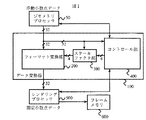•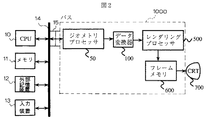•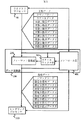••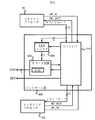•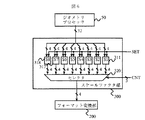•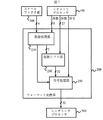••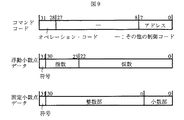•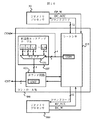•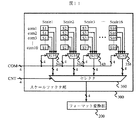••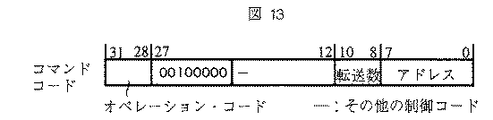•## Description


TECHNICAL FIELD OF THE INVENTION
The present invention relates to a graphics processing apparatus that performs graphics processing such as 3D graphics, and more particularly to a technique for speeding up the processing.

[Prior art]
As a conventional graphics processing apparatus, for example, an apparatus described in JP-A-8-161525 is known.

These conventional graphics processing devices generally include a geometry section for performing geometric calculations such as coordinate transformation and clipping processing, and a rendering section for converting a figure such as a straight line or a triangle into pixels and performing drawing processing. You.

In such a graphics processing apparatus, when an image is generated by projecting the 3D graphics data from various 3D graphics data, first, the geometry unit performs a perspective transformation to a two-dimensional coordinate of a three-dimensional shape and a clipping process. Calculate the brightness of the light source, etc. Then, in the rendering unit, the geometry unit expands data such as straight lines and triangles, which are graphic data obtained by projecting the three-dimensional shape into two-dimensional coordinates, into pixels, An operation such as alpha blending is performed, and the image is stored in the frame memory.

In such a graphics processing device, the geometry section is usually realized as one processor chip, and the operation in the geometry section is performed by floating each data so that the range of the numerical value is limited and the operation precision is not reduced. It is treated as a decimal number.

On the other hand, the rendering unit is also usually realized as one processor chip, but the operation is performed by treating each data as a fixed-point number. This is because it is difficult to perform the operation performed by the rendering unit using floating point numbers in terms of the circuit scale and the operation speed.

Further, the bit position of the decimal point of the fixed-point number handled in the rendering unit usually differs depending on the type of data. This is because the accuracy required for generating a high-quality image differs for each data type.

As described above, in the conventional graphics processing apparatus, the number representation of data handled by the two differs, such as the geometry section handles data using floating-point numbers and the rendering section handles data using fixed-point numbers. Therefore, conventionally, in the geometry unit, the data after the operation is converted from the floating-point number to the fixed-point number, and the data converted to the fixed-point number is passed to the rendering unit. For example, in the device described in the above-mentioned Japanese Patent Application Laid-Open No. 8-161525, a number obtained by multiplying a floating point number by 4096 is represented by an integer expression, so that data represented by the integer expression is converted to a fixed-point 12-bit fixed-point number. Numbers are correctly represented as numbers.

[Problems to be solved by the invention]
As described above, in the conventional graphics processing device, it was necessary to convert a floating point number into a fixed point number in the geometry part. In addition, the contents of the conversion to the fixed-point number differ for each type of data because the bit position of the decimal point of the fixed-point number required for rendering differs depending on the type of data.

Here, performing the conversion from the floating-point number to the fixed-point number in the geometry part in this way increases the load on the geometry part, and during this time, the arithmetic unit in the geometry part converts the floating-point number to the fixed-point number. , The geometry unit cannot perform original processing such as coordinate conversion, and lowers the throughput of the entire graphics processing apparatus. When the geometry section is operated by a program, a procedure for converting a floating-point number into a fixed-point number, which differs for each type of data, must be defined in the program.

Therefore, an object of the present invention is to provide a graphics processing device capable of reducing a reduction in processing speed required for converting a floating-point number to a fixed-point number.

[Means for Solving the Problems]
To achieve the above object, the present invention provides a processor that outputs graphic data including a floating-point data string representing information specifying a graphic to be drawn in a floating-point format,
A rendering unit that expands the figure into pixels and outputs the figure according to drawing data including a fixed-point data string representing the contents of the figure to be drawn in a fixed-point format,
A floating point data included in the graphic data output by the processor, converted to fixed-point data, comprising a data converter that outputs to the rendering unit as the drawing data,
The data converter includes a decimal point position changing unit that changes a bit position of a decimal point of the fixed point data for converting the floating point data.

According to such a graphic processing device according to the present invention, a data converter for converting floating-point data to fixed-point data is provided between the processor and the rendering unit. And floating-point data to fixed-point data, enabling high-speed graphics processing. In addition, since the bit position of the decimal point of the fixed-point data to be converted can be changed for each individual floating-point data, it is possible to cope with various kinds of data.

BEST MODE FOR CARRYING OUT THE INVENTION
Hereinafter, an embodiment of a graphics processing device according to the present invention will be described.

First, a first embodiment will be described.

FIG. 1 shows a configuration of a graphics processing apparatus according to the present embodiment.

As illustrated, the graphics processing device according to the present embodiment includes a geometry processor 50, a data converter 100, a rendering processor 500, and a frame memory 600.

The data converter 100 includes a control unit 400, a scale factor unit 300, and a format conversion unit 200.

Here, FIG. 2 shows a configuration of a computer to which the graphics processing device shown in FIG. 1 is applied.

As shown in the drawing, in the computer, a CPU 10, a memory 20, an external storage device 12, and an input device 13 such as a keyboard, a mouse, and a pen input tablet are connected via a bus 14. Further, a slot 15 for mounting an expansion card is provided on the bus 14, and the graphics processing apparatus 100 realized as an expansion card is connected to the bus 14 via this slot. Also, a CRT 700 as a display device is connected to the graphics processing device.

In such a configuration, the CPU 10 executes the application read from the memory 14 and generates graphics commands such as triangles and straight lines, and graphics data including vertex coordinates, normal vectors, and textures given by floating-point numbers. , To the geometry processor 50 via the system bus.

The geometry processor 50 performs coordinate conversion, clipping processing, calculation of luminance for a light source, and the like on the graphics data, and outputs commands such as triangles and straight lines, coordinates and luminance data after the geometric processing, a scale factor and floating point data. The data is converted into graphic data composed of columns and output to the data conversion unit 100.

The data converter 100 passes drawing data obtained by converting the graphic data passed from the geometry processor 50 to a fixed-point data sequence to the rendering processor 500.

The rendering processor 500 develops data such as straight lines and triangles indicated by the drawing data obtained by converting the graphic data into fixed-point numbers by the data conversion unit 100 into pixels, and performs Z comparison, alpha blending, and the like with the frame memory 600. An operation is performed, and processing for storing pixels obtained as an operation result in the frame memory 600 is performed.

The frame memory 600 is constantly read, and the contents of the frame memory 600 are displayed on the CRT 700 as a display device.

The graphics processing device according to the present embodiment can be applied to various devices such as a game device, a computer having a configuration other than that shown in FIG. 2, and a word processor, in addition to the components shown in FIG.

Hereinafter, the details of the graphics processing apparatus will be described focusing on the data converter 100 which is the most characteristic part of the present invention.

First, graphic data input from the geometry processor 50 to the data converter 100 and drawing data output from the data converter 100 to the rendering processor 500 will be described.

As shown in FIG. 3, the graphic data provided from the geometry processor 50 to the data converter 100 includes a command code, scale data, and floating point data 1 to 8.

For example, the graphic data of a straight line is
The command code contains a straight line drawing command, transfer number 7 and address,
The scale data contains a scale factor used when converting floating-point data 1 to 7 to fixed-point data 1 to 7,
Floating point data 1 contains the starting point X coordinate of floating point data,
Floating point data 2 contains the Y coordinate of the starting point of the floating point data,
The floating point data 3 contains the X coordinate of the end point of the floating point data,
The floating point data 4 contains the X coordinate of the end point of the floating point data,
The floating point data 5 contains the luminance R of the floating point data,
The floating point data 6 contains the luminance G of the floating point data,
The floating point data 7 contains the luminance B of the floating point data.

As shown in FIG. 3, the data converter 100 receives such graphic data, and renders command codes and fixed-point data 1 to 8 obtained by converting floating-point data 1 to 8 to fixed-point data as drawing data. Pass to processor 500.

For example, drawing data obtained by converting the straight line graphic data is as follows:
The command code contains a straight line drawing command, transfer number 7 and address,
The fixed point data 1 contains the starting point X coordinate of the fixed point data,
The fixed point data 2 contains the Y coordinate of the start point of the fixed point data,
The fixed point data 3 contains the X coordinate of the end point of the fixed point data,
The fixed point data 4 contains the X coordinate of the end point of the fixed point data,
Fixed point data 5 contains the luminance R of the fixed point data,
The fixed point data 6 contains the luminance G of the fixed point data,
The brightness B of the fixed-point data is included in the fixed-point data 7.

Here, FIG. 4 shows a format of the input / output data of the data converter 100 described above.

The command code includes an operation code, other control codes, the number of transfers, and an address. The operation code describes the operation content instructed to the rendering processor 500, the transfer number describes the number of fixed-point numbers to be passed to the rendering processor 500, and the address describes the register of the rendering processor 500 to which the graphic data is written. The other control codes store control information to be transmitted from the geometry processor 50 to the rendering processor 500 or the data converter 100 as necessary.

Next, there are scale data from Scale1 to Scale8, and each Scale has a scale factor. Scale1 corresponds to the floating-point data 1 and the fixed-point data 1 in FIG. 3, Scale2 corresponds to the floating-point data 2 and the fixed-point data 2, and so on for Scale3 to Scale8. Here, for example, if the value of Scale1 is 3, this indicates that the position of the decimal point of the fixed-point data 1 passed from the data converter 100 to the rendering processor 500 is the third bit from the least significant bit. Is 5, the position of the decimal point of the fixed-point data 2 passed from the data converter 100 to the rendering processor 500 is set to the fifth bit from the least significant bit.

Next, the floating-point data is a well-known IEEE single-precision floating-point representation, and includes 1 bit for a sign, 8 bits for an exponent, and 23 bits for a mantissa.

Finally, the fixed-point data includes a sign, an integer part, and a decimal part, and the bit position of the decimal point changes according to the scale factor.

Hereinafter, the operation of the data converter 100 will be described.

In the data converter 100, the control unit 400 decodes a command code of graphic data including a command code, scale data, and floating-point data generated by the geometry processor 50, and converts the floating-point data to fixed-point data. A set of scale factors and an instruction on which scale factor to use are given to the scale factor unit 300.

The scale factor unit 300 operates in accordance with a set of scale factor strings from the control unit 400 and a control signal for instructing which scale factor to use, and gives the format conversion unit 200 a scale factor, that is, a bit position of a decimal point of a fixed-point number. give.

The format conversion unit 200 converts the floating-point data from the geometry processor 50 into fixed-point data so as to correspond to the scale factor received from the scale factor unit 300, and supplies the fixed-point data to the rendering processor 500.

Hereinafter, details of each unit will be described.

FIG. 5 shows a configuration of the control unit 400.

The control unit 400 manages the state of the data converter 100 and controls the transfer of graphics and drawing data to and from the geometry processor 50 and the rendering processor 500, and the number of fixed-point data to be passed to the rendering processor 500. And a LEN register 430 for storing the number of transfers included in the command code.

The state managed by the sequencer 410 includes a state where the data converter 100 receives a command code from the geometry processor 50, a state where the data converter 100 receives scale data, and a state where the data converter 100 receives floating point data 1 to 8.

While receiving the command code, the sequencer 410 issues an instruction to set the number of transfers in the command code to the LEN register 430, and instructs the counter circuit 420 of the CNT register indicating the number of fixed-point data passed to the rendering processor 500. , An initialization instruction, a command code write request DC_W signal of the rendering data to the rendering processor 500, and the command code is passed to the rendering processor 500. Then, when a signal RP_ACC signal indicating that the drawing data command code has been received is returned from the rendering processor 500, a signal DC_ACC signal indicating that the command code has been received is output to the geometry processor 50, and the state is received as scale data. Transition to the state.

Next, while receiving the scale data, the sequencer 410 issues an instruction SET signal for writing the scale data to the scale factor section 300, and issues a signal DC_ACC signal indicating that the scale data has been accepted to the geometry processor 50. To the state receiving floating point data 1 to 8.

Next, while receiving the floating-point data 1 to 8, the sequencer 410 issues a request DC_W signal for writing fixed-point data to the rendering processor 500, and a signal RP_ACC indicating that fixed-point data has been received from the rendering processor 500. When the signal is returned, the signal DC_ACC indicating that the graphic data has been received is output to the geometry processor 50.

When receiving an instruction from the counter circuit 420 to return to a state in which the command code is received while the sequencer 410 is receiving the floating-point data 1 to 8, the sequencer 410 transits to a state in which the command code is received. Otherwise, the operation of receiving the floating point data 1 to 8 is repeated.

Here, the counter circuit 420 outputs an instruction to return to a state in which the command code is received, as follows.

As described above, the LEN register 430 is a register that stores the number of transfers in the command code. The LEN register 430 stores the number of transfers in the command code in accordance with an instruction to set the number of transfers in the command code, and stores the stored value in the counter circuit 420. Output. The counter circuit 420 includes a CNT register and a comparator. When the CNT register receives an initialization instruction from the sequencer 410, the register value is initialized to 0, the value of the CNT register is given to the scale factor unit 300, and the sequencer 410 receives floating point data 1 to 8 in a state where it is received. When the signal RP_ACC, which has received the rendering data fixed-point data from the rendering processor 500, returns, the value of the CNT register is incremented. Then, the comparator compares the value of the incremented CNT register with the value of the LEN register, and when the value of the CNT register matches the value of the LEN register, instructs the sequencer 410 to return to a state in which the command code has been received. give.

Next, FIG. 6 shows a configuration of the scale factor unit 300.

As shown, the scale factor unit 300 includes registers S1 311, S2 312, S3313, S4 314, S5 315, S6 316, S7 317, S8 318 and a selector.

Registers S1 311 to S8 318 are registers for holding a scale factor corresponding to each of floating point data 1 to 8, and when a write instruction SET signal is received from the control unit 400, a 32-bit scale passed from the geometry processor 50. The data is distributed and written in four bits.

The selector 320 selects one of the values of the registers S1 311 to S8 318 based on the counter value CNT signal input from the control unit 400, and outputs the selected value to the format conversion unit 200. Thus, a corresponding scale factor can be given to the format conversion unit 200 for each of the floating point data 1 to 8 of the graphic data.

Next, FIG. 7 shows a configuration of the format conversion unit 200.

As shown, the format conversion unit 200 includes an exponent processing unit 210 that calculates a shift amount from a scale factor and an exponent of a floating-point number, and a mantissa shift that shifts a mantissa of a floating-point number to the right to convert it into a positive fixed-point number. It comprises a unit 230 and a sign processing unit 250 that converts a positive fixed-point number obtained by the mantissa shift unit into a negative fixed-point number if the sign of the floating-point number is negative.

The exponent processing unit 210 adds the value of the scale factor output from the scale factor unit 300 and the exponent of a floating-point number, subtracts an offset value of 127 from the addition result, performs offset correction, and performs data offset correction. By extracting only the lower 5 bits and performing bit inversion, the shift amount of the mantissa shift unit 230 is obtained.

Here, the reason why the offset value of 127 is subtracted from the addition result is that an offset of 127 is added to the exponent of the floating-point number according to the IEEE standard. Further, the reason why only the lower 5 bits are extracted is that only a 5-bit information is required for the shift amount in order to convert to a 32-bit fixed-point number. The reason why the bit inversion is performed is to convert the value of the exponent intended for left shift to right shift.

Further, if the value obtained by adding the value of the scale factor output from the scale factor unit 300 and the exponent of the floating-point number is less than the constant 127, the exponent processing unit 210 may convert the value to a 32-bit fixed-point number. Is too small to represent, so that processing for outputting an instruction to clamp the data of the fixed-point number to 0 to the sign processing unit 250 is also performed.

Next, the mantissa shift section 230 inserts 1 into the most significant bit, inserts 23 bits of the mantissa of the floating-point number into bits 30 to 8, and calculates a 32-bit coefficient with bits 7 to 0 set to 0. Is shifted to the right by the shift amount obtained by the exponent processing unit 210, and is converted into a positive fixed-point number. The reason why 1 is inserted in the most significant bit of the 32-bit coefficient is that the most significant 1 of the mantissa is omitted in the IEEE standard.

Next, if the sign of the floating-point number is minus, the sign processing unit 250 converts the positive fixed-point data obtained by the mantissa shift unit 230 into negative fixed-point data, When the instruction is issued, the fixed-point data is set to 0, and the fixed-point data is passed to the rendering processor 500.

Here, an example in which a floating point number of 2.625 is converted into a fixed point number having a decimal part of 3 bits will be described below.

A floating point number of 2.625 has a sign bit of (0) 2 , The index is (10000000) 2 ,
The mantissa is (0111000000000000000000000) 2 Is represented by

When the exponent processing section 210 adds the bit position of the decimal point and the exponent (10000011) 2 When offset correction is performed by subtracting 127, (00000100) 2 become.

Then, when the lower 5 bits are extracted, (00100) 2 become,
When bit inversion is performed (11011) 2 , And the shift amount becomes 27.

The 32-bit coefficient of the mantissa shift unit 230 is
(10101000000000000000000000000000000) 2 Becomes
If this is shifted right by 27 bits,
(00000000000000000000000000000101101) 2 And a positive fixed-point number can be obtained.

The lower 4 bits or more as an integer part indicate 2 and the lower 3 bits as a decimal part are:
0.625 is indicated.

In the sign processing unit 250, the sign bit is (0) 2 Since it indicates positive, it is passed to the rendering processor 500 without converting the positive fixed-point number into a negative fixed-point number.

The first embodiment of the graphics processing device according to the present invention has been described above.

Hereinafter, a second embodiment of the graphics processing device according to the present invention will be described.

The overall configuration of the graphic processing device according to the second embodiment is the same as the configuration shown in FIG. 1 above, and can be applied to a computer as shown in FIG.

FIG. 8 shows graphic data input from the geometry processor 50 to the data converter 100 and drawing data output from the data converter 100 to the rendering processor 500 in the second embodiment.

As shown in the figure, the graphic data of the second embodiment is obtained by omitting the scale data from the graphic data input from the geometry processor 50 to the data converter 100 in the first embodiment.

Next, FIG. 9 shows an input / output data format of the data converter 100.

As shown, the formats of the floating point data, fixed point data, and command data are the same as those described in the first embodiment.

Here, the configuration of the graphic data output from the geometry processor 50 can be specified from the operation code of the command code.

For example, if the operation code is (0001) 2 In the case of, this means a command for drawing a straight line. In this case, it is determined that the floating point data 1 to 7 indicate the starting point X, Y coordinate, the ending point X, Y coordinate, and the luminance R, G, B. ing. Also, (0010) 2 In the case of, this means a command for drawing a triangle, whereas floating point data 1 to 5 are determined to indicate vertices X, Y coordinates, and luminances R, G, and B. When one triangle is displayed on the CRT 700, graphic data of the triangle is given to the rendering processor 500 three times.

In this manner, the type of each floating-point data following the command code including the operation code is determined for the value of the operation code. Therefore, in the second embodiment, in the data converter 100, for example, the operation code is (0001) 2 In the case of, the floating point data 1 starting point X coordinate is converted to fixed point data 1 in which the decimal point is located at the third bit from the least significant bit, and the floating point data 2 starting point Y coordinate is converted to the second bit from the least significant bit. Is converted to the fixed-point data 2 located at, and the operation code is (0010). 2 In the case of, the X coordinate of the floating point data 1 vertex is converted to the fixed point data 1 whose decimal point is located at the fourth bit from the least significant bit, and the Y coordinate of the floating point data 2 vertex is located at the second bit from the least significant bit. According to the operation code and the position of the floating-point data, the bit position of the decimal point of the fixed-point data to be converted from the floating-point data is determined according to a preset correspondence. Accordingly, each floating-point data is converted into a fixed-point number having a bit position of a decimal point according to the type of the data without receiving scale data from the geometry processor unit 50 as in the first embodiment.

Hereinafter, the details of the data converter 100 that performs such conversion will be described. The overall configuration of the data converter 100 is the same as that shown in FIG. 1 and includes a control unit 400, a scale factor unit 300, and a format conversion unit 200.

FIG. 10 shows the configuration of the control unit 400.

As illustrated, the control unit 400 includes a sequencer 415 that manages the state of the data converter 100 and controls the transfer of graphics and drawing data to the geometry processor 50 and the rendering processor 500, and data to be passed to the rendering processor 500. And a transfer number lookup table 435 for detecting the number of transfers in the command code from the operation code.

The state managed by the sequencer 415 includes a state in which the data converter receives a command code from the geometry processor 50 and a state in which the data converter receives floating point data 1 to 8.

While receiving the command code, the sequencer 415 stores the operation code of the command code in the COM register, outputs the content of the operation code to the transfer number lookup table 435, and instructs the counter circuit 420 to initialize the CNT register. And sends a rendering data command code write request DC_W signal to the rendering processor 500, and passes the command code to the rendering processor 500. When a signal RP_ACC indicating that the drawing data command code has been received is returned from the rendering processor 500, a signal DC_ACC indicating that the graphic data has been received is output to the geometry processor 50, and the state is changed from the floating-point data 1 to 8 Transits to the state in which

Next, when receiving the floating-point data 1 to 8, the sequencer 415 supplies the value of the COM register in which the operation code is stored to the scale factor unit 300, and the request DC_W signal for writing the fixed-point data to the rendering processor 500. When a signal RP_ACC indicating that fixed-point data has been received is returned from the rendering processor 500, a signal DC_ACC indicating that floating-point data has been received is output to the geometry processor 50. When receiving an instruction from the counter circuit 420 to return to the state in which the command code is received while the sequencer 415 is receiving floating point data 1 to 8, the sequencer 415 transitions to the state in which the command code is received. Otherwise, the operation of receiving the floating point data 1 to 8 is repeated.

In the transfer number lookup table 435, the value of the transfer number of the fixed-point data is registered from L1 to L16 for each operation code, and one of the values is registered in accordance with the value of the COM register output from the sequencer 415. , And are supplied to the counter circuit 420 by the selector 436.

For example, the transfer number 7 is registered in L1 and the transfer number 5 is registered in L2, and the value operation code of the COM register is (0001) 2 In the case of, the transfer number 7 is given to the counter circuit 420, and the value of the COM register is (0010) 2 In the case of, the transfer number 5 is given.

The counter circuit 420 includes a CNT register and a comparator. When the CNT register receives an initialization instruction from the sequencer 415, the register value is initialized to 0, the value of the CNT register is given to the scale factor section 300, and the sequencer 415 receives floating point data 1 to 8 in a state where it is received. When the signal RP_ACC that has received the drawing data fixed-point data from the rendering processor 500 returns, the value of the CNT register is incremented. The comparator compares the incremented value of the CNT register with the number of transfers sent from the transfer number look-up table 435, and if the values match, returns to the state in which the sequencer 415 has received the command code. Give instructions.

FIG. 11 shows the configuration of scale factor section 300.

The scale factor unit 300 has a table for registering scale factors from Scale1 to Scale16 for each operation code.

One of a plurality of scale factors stored in the table of Scale 1 is selected by the selector 341 in accordance with the value COM signal of the COM register output from the control unit 400, and is output to the selector 360. Similarly, for Scale2 to Scale16, the scale factor corresponding to the value COM signal of the COM register is selected one by one and output to the selector 360.

Next, each value from Scale1 to Scale16 selected by each of the selectors 341 to 356 is selected one by one by the selector 360 by the counter value CNT signal input from the control unit, and is output to the format conversion unit 200. Thus, a corresponding scale factor can be given to the format conversion unit 200 for each of the floating point data 1 to 8 of the graphic data.

The format converter 200 is the same as the format converter 200 of the first embodiment described above.

As above, the second embodiment of the present invention has been described.

In the second embodiment, the contents of the transfer number lookup tables L1 to L16 storing the transfer numbers determined corresponding to the operation codes and the scale factor tables S1 to S16 are, for example, the CPU 10 of FIG. An arbitrary value may be set from the geometry processor 50. By doing so, it is possible to appropriately change the format of the graphic data.

Hereinafter, a third embodiment of the present invention will be described.

In the third embodiment, in the graphic processing apparatus according to the first embodiment, the data converter 100 does not convert floating-point data to fixed-point data, but renders the rendering processor 500 in the format as received from the geometry processor 50. It has a function to pass to.

Such a function is performed when there is data to be processed as floating point data even in the rendering processor 500, and as shown in FIG. 12, not only floating point data but also command data is included in data following command data as operation data. When adopting a format in which data including a code or the like exists, it is effective to pass such data to the rendering processor 500 as it is.

FIG. 13 shows the format of the command data in this case.

As shown in FIG. 13, in the third embodiment, a conversion control code indicating data that is not converted to a fixed point is added to other control codes following the operation code of the command code.

(00100000) in the figure 2 Is the value (1) 2 , That is, the third data from the front indicates that the data should be output to the rendering processor 500 without being converted into fixed-point data in the data converter 100.

Hereinafter, the data converter 100 according to the present embodiment will be described.

The overall configuration of the data converter 100 is the same as that shown in FIG. 1 and includes a control unit 400, a scale factor unit 300, and a format conversion unit 200. The control unit 400 and the scale factor unit 300 may be the same as those described in the first embodiment.

FIG. 14 shows the configuration of the remaining format conversion unit 200.

As shown, the format conversion unit 210 according to the present embodiment is obtained by adding a control code processing unit 290 and a selector 291 to the configuration of the format conversion unit 200 shown in FIG.

When the sequencer 410 of the control unit 400 receives the command code, the control code register in the control code processing unit 290 stores the conversion control code in the command code and other control codes.

The control code processing unit 290 controls the selector 291 so that the selector 291 normally selects the fixed-point data output from the code processing unit 250, but the value output from the counter circuit 420 of the control unit 400 is stored in the register. Value of position indicating that stored conversion control code does not convert data (conversion control code is (00100001) 2 If it becomes the same as 3 and 8), the selector 291 controls the selector 291 so as to select the fixed-point data received from the geometry processor 50.

Hereinabove, the third embodiment of the present invention has been described.

Note that, in the graphics processing device described in the second embodiment, the format conversion unit 200 of the data converter 100 is configured as shown in FIG. It can be applied to the second embodiment in the same manner as the application.

According to the embodiments described above, the data converter 100 is provided between the geometry processor 50 and the rendering processor 500, and the processing of the geometry processor 10 and the conversion of floating-point data to fixed-point data are performed in a pipeline manner. Therefore, high-speed graphics processing can be performed. Further, since the bit position of the decimal point can be arbitrarily controlled for each data type, and conversion from a floating-point number to a fixed-point number is not performed, a wide variety of data can be handled.

【The invention's effect】
As described above, according to the present invention, it is possible to provide a graphics processing apparatus capable of reducing a reduction in processing speed required for converting a floating-point number to a fixed-point number.
[Brief description of the drawings]
FIG. 1 is a block diagram illustrating a configuration of a graphics processing device.
FIG. 2 is a block diagram illustrating a configuration of a computer to which the graphics processing device is applied.
FIG. 3 is a diagram showing input / output data of a data converter according to the first embodiment of the present invention.
FIG. 4 is a diagram showing an input / output data format of the data converter according to the first embodiment of the present invention.
FIG. 5 is a block diagram illustrating a configuration of a control unit according to the first embodiment of the present invention.
FIG. 6 is a block diagram illustrating a configuration of a scale factor unit according to the first embodiment of the present invention.
FIG. 7 is a block diagram illustrating a configuration of a format conversion unit according to the first embodiment of the present invention.
FIG. 8 is a diagram showing input / output data of a data converter according to a second embodiment of the present invention.
FIG. 9 is a diagram showing an input / output data format of a data converter according to a second embodiment of the present invention.
FIG. 10 is a block diagram illustrating a configuration of a control unit according to a second embodiment of the present invention.
FIG. 11 is a block diagram illustrating a configuration of a scale factor unit according to a second embodiment of the present invention.
FIG. 12 is a diagram showing input / output data of a data converter according to a third embodiment of the present invention.
FIG. 13 is a diagram showing a format of command data according to a third embodiment of the present invention.
FIG. 14 is a block diagram illustrating a configuration of a format conversion unit according to a third embodiment of the present invention.
[Explanation of symbols]
10 CPU, 50 Geometry processor, 100 Data converter, 200 Format converter, 210 Exponent processor, 230 Mantissa shift unit, 250 Code Processing unit, 290: control code processing unit, 291: selector, 300: scale factor unit, 311 to 318: registers S1 to S8, 320: selector, 341 to 356: selector , 360... Selector. 400 control unit, 410 sequencer, 415 sequencer, 435 transfer number lookup table, 436 selector, 420 counter circuit, 430 LEN register, 500 ... Render rig processor, 600 ... Frame memory, 700 ... CRT,

## Claims (5)

1. A processor for outputting graphic data including a floating-point data string representing information for specifying a graphic to be drawn in a floating-point format;
A rendering unit that expands the figure into pixels and outputs the figure according to drawing data including a fixed-point data string representing the contents of the figure to be drawn in a fixed-point format,
A data converter that converts floating-point data included in the graphic data output by the processor to fixed-point data and outputs the drawing data to the rendering unit,
The data converter has a decimal point position changing means for changing the bit position of the decimal point of the fixed point data for converting the floating point data,
The processor outputs, as the graphic data, scale factor data specifying a bit position of a decimal point of fixed-point data to be converted with respect to each floating-point data, together with the floating-point data sequence,
The decimal point position changing means of the data converter determines the bit position of the decimal point of the fixed-point data for converting each floating-point data, for the floating-point data, the scale factor data included in the graphic data output by the processor. A graphics processing device wherein a bit position of a designated decimal point is set.
2. A processor for outputting graphic data including a floating-point data string representing information for specifying a graphic to be drawn in a floating-point format;
A rendering unit that expands the figure into pixels and outputs the figure according to drawing data including a fixed-point data string representing the contents of the figure to be drawn in a fixed-point format,
A data converter that converts floating-point data included in the graphic data output by the processor to fixed-point data and outputs the drawing data to the rendering unit,
The data converter has a decimal point position changing means for changing the bit position of the decimal point of the fixed point data for converting the floating point data,
The processor outputs, as the graphic data, a command representing a type of a graphic specified by the floating-point data sequence, together with the floating-point data sequence,
The data converter includes a scale factor table that stores a bit position of a decimal point of the fixed-point data for converting each floating-point data included in the graphic data in correspondence with each of the commands,
The decimal point position changing means of the data converter sets the bit position of the decimal point of the fixed-point data for converting each floating-point data to the scale factor table corresponding to the command included in the same graphic data as the floating-point data. A graphics processing device, wherein the position of the decimal point is stored in the graphics processing device.
3. The graphics processing device according to claim 2 , wherein
The scale factor table of the data converter stores the bit position of the decimal point of the fixed-point data for converting each floating-point data included in the graphic data, in correspondence with the position of the floating-point data in the graphic data and each command. With a scale factor table
The decimal point position changing means of the data converter sets a bit position of a decimal point of the fixed-point data for converting each floating-point data to the command and the graphic data of the floating-point data included in the same graphic data as the floating-point data. A graphics processing device, wherein a bit position of a decimal point stored in the scale factor table corresponding to a middle position is set.
4. The graphics processing device according to claim 1, 2 or 3 ,
The processor outputs, as the graphic data, a control code that specifies floating-point data that is not converted to fixed-point data among floating-point data included in the floating-point data string, together with the floating-point data string;
The graphics processing device, wherein the data converter does not convert floating-point data specified by a control code output from the processor into fixed-point data, but includes the data in the drawing data and outputs the data to the rendering unit. .
5. A graphics processing device according to claim 1, 2, 3, or 4 ,
A frame memory for storing pixels that the rendering unit has developed and output graphics,
A display device for sequentially reading and displaying each pixel stored in the frame memory.
JP01091897A 1997-01-24 1997-01-24 Graphics processing unit Expired - Fee Related JP3547277B2 (en)

## Priority Applications (1)

Application Number Priority Date Filing Date Title
JP01091897A JP3547277B2 (en) 1997-01-24 1997-01-24 Graphics processing unit

## Applications Claiming Priority (6)

Application Number Priority Date Filing Date Title
JP01091897A JP3547277B2 (en) 1997-01-24 1997-01-24 Graphics processing unit
US09/009,588 US6049343A (en) 1997-01-20 1998-01-20 Graphics processing unit and graphics processing system
CA 2227531 CA2227531C (en) 1997-01-20 1998-01-20 Graphics processing unit and graphics processing system
CA002418916A CA2418916C (en) 1997-01-20 1998-01-20 Graphics processing unit and graphics processing system
US10/391,235 US20030163502A1 (en) 1997-01-20 2003-03-19 Graphics processing unit and graphics processing system
US11/484,812 US20060250397A1 (en) 1997-01-20 2006-07-12 Graphics processing unit and graphics processing system

## Publications (2)

Publication Number Publication Date
JPH10207688A JPH10207688A (en) 1998-08-07
JP3547277B2 true JP3547277B2 (en) 2004-07-28

# Family

## Family Applications (1)

Application Number Title Priority Date Filing Date
JP01091897A Expired - Fee Related JP3547277B2 (en) 1997-01-24 1997-01-24 Graphics processing unit

## Country Status (1)

JP (1) JP3547277B2 (en)

## Families Citing this family (1)

* Cited by examiner, † Cited by third party
Publication number Priority date Publication date Assignee Title
US7236995B2 (en) * 2002-12-27 2007-06-26 Arm Limited Data processing apparatus and method for converting a number between fixed-point and floating-point representations

## Also Published As

Publication number Publication date
JPH10207688A (en) 1998-08-07

## Similar Documents

Publication Publication Date Title
JP6487097B2 (en) Perform rounding according to instructions
US5892517A (en) Shared access texturing of computer graphic images
US7518615B1 (en) Display system having floating point rasterization and floating point framebuffering
EP1725989B1 (en) Register based queuing for texture requests
US6573846B1 (en) Method and apparatus for variable length decoding and encoding of video streams
TWI250785B (en) Image rendering device and image rendering method
US6697076B1 (en) Method and apparatus for address re-mapping
US6784888B2 (en) Method and apparatus for executing a predefined instruction set
DE60018078T2 (en) Setting conditional values in a computer
US6385713B2 (en) Microprocessor with parallel inverse square root logic for performing graphics function on packed data elements
US5594854A (en) Graphics subsystem with coarse subpixel correction
US4467412A (en) Slave processor with clock controlled by internal ROM &amp; master processor
JP3778517B2 (en) Method and apparatus for color space conversion using blend logic
KR100239029B1 (en) Result normalizer and method of operation
KR101138378B1 (en) Image processor
US4862392A (en) Geometry processor for graphics display system
US5025407A (en) Graphics floating point coprocessor having matrix capabilities
US6891544B2 (en) Game system with graphics processor
KR101349171B1 (en) 3-dimensional graphics accelerator and method of distributing pixel thereof
Segal et al. The OpenGL graphics system: A specification (version 1.1)
US8106914B2 (en) Fused multiply-add functional unit
KR100362704B1 (en) Image data generation method and image data processing apparatus and recording medium
US6014144A (en) Rapid computation of local eye vectors in a fixed point lighting unit
US6825843B2 (en) Method and apparatus for loop and branch instructions in a programmable graphics pipeline
US4648049A (en) Rapid graphics bit mapping circuit and method

## Legal Events

Date Code Title Description
TRDD Decision of grant or rejection written
A01 Written decision to grant a patent or to grant a registration (utility model)

Free format text: JAPANESE INTERMEDIATE CODE: A01

Effective date: 20040406

A61 First payment of annual fees (during grant procedure)

Free format text: JAPANESE INTERMEDIATE CODE: A61

Effective date: 20040413

FPAY Renewal fee payment (event date is renewal date of database)

Free format text: PAYMENT UNTIL: 20090423

Year of fee payment: 5

FPAY Renewal fee payment (event date is renewal date of database)

Free format text: PAYMENT UNTIL: 20090423

Year of fee payment: 5

FPAY Renewal fee payment (event date is renewal date of database)

Free format text: PAYMENT UNTIL: 20100423

Year of fee payment: 6

FPAY Renewal fee payment (event date is renewal date of database)

Free format text: PAYMENT UNTIL: 20110423

Year of fee payment: 7

FPAY Renewal fee payment (event date is renewal date of database)

Free format text: PAYMENT UNTIL: 20120423

Year of fee payment: 8

FPAY Renewal fee payment (event date is renewal date of database)

Free format text: PAYMENT UNTIL: 20120423

Year of fee payment: 8

FPAY Renewal fee payment (event date is renewal date of database)

Free format text: PAYMENT UNTIL: 20130423

Year of fee payment: 9

FPAY Renewal fee payment (event date is renewal date of database)

Free format text: PAYMENT UNTIL: 20140423

Year of fee payment: 10

LAPS Cancellation because of no payment of annual fees